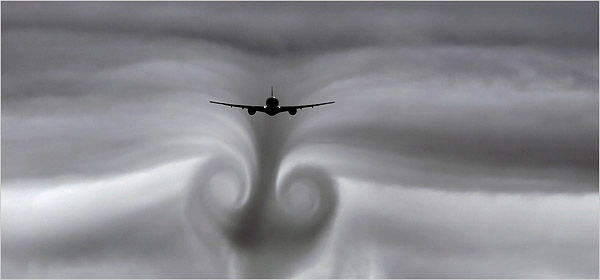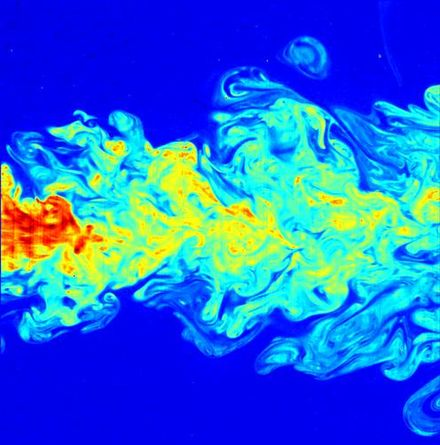# Turbulence simulation

Hi

I am thinking to develop a turbulence simulation in grasshopper. Any thoughts about how to start?A paper from MIT

What will your simulation of turbulence be used for? Will it be used for engineering purposes? Or will it be used for visual purposes such as shapes for use in a design?

The phenomena in the image below is primarily large scale vorticity, not turbulence…

My intention is Design usage. I guess engineering has its own professional software.

Accurate modeling of turbulence requires very intensive computations with software created for that purpose. Usually for engineering purposes only the average properties of turbulence are calculated.

it is possible to create patterns or textures very similar to turbulence using fractals. At one time there was considerable research into modeling turbulence using fractals. My understanding is while the results frequently looked similar they were not generally not close enough to be used for engineering.

There is a similar approach by Zaha Hadid Architects that might be very interesting to you: https://www.archdaily.com/801031/mathematics-the-winton-gallery-zaha-hadid-architects

1 Like

This is a very nice Video on this topic

3 Likes

Hello
You could look at that

I feel the form is more like minimal surface.

Yes it is. But it´s derived by a plane turbulance.

So they tracked plane turbulence to get curves, and then from the curves generate minimal surface as spacial form.

Exactly. that´s how I understood it.

have look at this. I was astonishing by the turbulence visual effect when watching her made it so easily. But her voice is bit hoarse.

1 Like

how did you make this?

Hello
it is written, I reuse the script from Long Nguyen. I mustn’t have done lot of changes !!
Initial script still there

``````private void RunScript(Polyline pl, int n, ref object A)
{
int size = 512;
SmokeSimulator2D s = new SmokeSimulator2D(size);
for (int i = 0; i < pl.Count - 1; i++)
{

}
for (int i = 0; i < n; i++)
{
s.Update();
}

A = s.GetMesh();

}

public class SmokeSimulator2D
{
// For more info and explanation of the algorithm, see "Real-Time Fluid Dynamics for Games" by Jos Stam

private float[] u; // Velocities in X direciton
private float[] v; // Velocities in Y direciton
private float[] d; // Smoke densities
private int gridSize; // Grid size
private Mesh displayMesh; // Mesh with vertex colors for display
private System.Drawing.Color[] colors; // vertex colors of the display mesh
public BoundingBox BoundingBox; // Bounding box for display
private float dt = 0.5f; // Timestep

private int addSmokeIndex = -1; // The index of the grid cell where smoke should be created (with mouse click and hold)

public SmokeSimulator2D(int gridSize)
{
this.gridSize = gridSize;
u = new float[gridSize * gridSize];
v = new float[gridSize * gridSize];
d = new float[gridSize * gridSize];

displayMesh = new Mesh();

for (int j = 0; j < gridSize; j++)
for (int i = 0; i < gridSize; i++)

for (int j = 0; j < gridSize - 1; j++)
for (int i = 0; i < gridSize - 1; i++)
displayMesh.Faces.AddFace(idx(i, j), idx(i + 1, j), idx(i + 1, j + 1), idx(i, j + 1));

colors = new Color[gridSize * gridSize];
BoundingBox = new BoundingBox(-1.0, -1.0, -1.0, gridSize + 1.0, gridSize + 1.0, 1.0);
}

public void AddSmoke(Point3d start, Point3d end)
{
// This function increase the smoke density and velocity at every grid cell along the line from start point to end point

Vector3d velocity = 30.0 * (end - start);
int i0 = (int) Math.Round(start.X);
int j0 = (int) Math.Round(start.Y);

int i1 = (int) Math.Round(end.X);
int j1 = (int) Math.Round(end.Y);

float count =
Math.Abs(end.X - start.X) > Math.Abs(end.Y - start.Y)
? Math.Abs(i1 - i0)
: Math.Abs(j1 - j0);

for (int k = 0; k <= count; k++)
{
float factor = k / count;
int i = (int) (factor * (i1 - i0) + i0);
int j = (int) (factor * (j1 - j0) + j0);
if (i <= 0 || i >= gridSize - 1 || j <= 0 || j >= gridSize - 1) return;
u[addSmokeIndex] += (1.0f - factor) * (float) velocity.X * dt;
v[addSmokeIndex] += (1.0f - factor) * (float) velocity.Y * dt;
}
}

public void Update()
{
float[] u0 = new float[gridSize * gridSize];
float[] v0 = new float[gridSize * gridSize];

Project(u, v, u0, v0);
Swap(ref u0, ref u);
Swap(ref v0, ref v);

float[] d0 = new float[gridSize * gridSize];
Swap(ref d0, ref d); Diffuse(d, d0, 0.3f);
Swap(ref d0, ref d); Advect(d, d0, u, v);
}

private void Diffuse(float[] x, float[] x0, float diffuseRate)
{
float temp1 = dt * diffuseRate;

{
for (int j = 1; j < gridSize - 1; j++)
x[idx(i, j)] = 0.98f * (x0[idx(i, j)] +
temp1 * (-x0[idx(i, j)] + 0.25f * (x0[idx(i - 1, j)] + x0[idx(i + 1, j)] + x0[idx(i, j - 1)] + x0[idx(i, j + 1)])));
});
}

void Advect(float[] d, float[] d0, float[] u, float[] v)
{
{
for (int j = 1; j < gridSize - 1; j++)
{
float x = i - dt * u[idx(i, j)];
float y = j - dt * v[idx(i, j)];
x = x < 0.5f ? 0.5f : (x > gridSize - 1.5 ? gridSize - 1.5f : x);
y = y < 0.5f ? 0.5f : (y > gridSize - 1.5 ? gridSize - 1.5f : y);
int i0 = (int) (x + 0.0001);
int j0 = (int) (y + 0.0001);

float tx = x - i0;
float ty = y - j0;
d[idx(i, j)] =
(1.0f - tx) * ((1.0f - ty) * d0[idx(i0, j0)] + ty * d0[idx(i0, j0 + 1)]) +
tx * ((1.0f - ty) * d0[idx(i0 + 1, j0)] + ty * d0[idx(i0 + 1, j0 + 1)]);
}
});
}

void Project(float[] u, float[] v, float[] p, float[] div)
{
{
for (int j = 1; j < gridSize - 1; j++)
{
div[idx(i, j)] = -0.5f * (u[idx(i + 1, j)] - u[idx(i - 1, j)] + v[idx(i, j + 1)] - v[idx(i, j - 1)]);
p[idx(i, j)] = 0.0f;
}
});

for (int k = 0; k < 15; k++)
{
{
for (int j = 1; j < gridSize - 1; j++)
p[idx(i, j)] = 0.25f *
(div[idx(i, j)] +
p[idx(i - 1, j)] + p[idx(i + 1, j)] +
p[idx(i, j - 1)] + p[idx(i, j + 1)]);
});

}

{
for (int j = 1; j < gridSize - 1; j++)
{
u[idx(i, j)] -= 0.5f * (p[idx(i + 1, j)] - p[idx(i - 1, j)]);
v[idx(i, j)] -= 0.5f * (p[idx(i, j + 1)] - p[idx(i, j - 1)]);
}
});
}

public void DrawGraphicsMesh(IGH_PreviewArgs args)
{

for (int i = 0; i < gridSize; i++)
for (int j = 0; j < gridSize; j++)
{
int c = ToColor(d[idx(i, j)]);
colors[idx(i, j)] = Color.FromArgb(128, c, c, c);
}

displayMesh.VertexColors.SetColors(colors);
args.Display.DrawMeshFalseColors(displayMesh);
}

public Mesh GetMesh()
{
for (int j = 0; j < gridSize; j++)
for (int i = 0; i < gridSize; i++)
displayMesh.Vertices.SetVertex(idx(i, j), i, j, 15.0 * d[idx(i, j)]);

return displayMesh;
}

public List<GH_Number> GetSmokeDensities()
{
List<GH_Number> ghNumbers = new List<GH_Number>();

foreach (float value in d)

return ghNumbers;
}

private int ToColor(float v)
{
return (int) (255 * Math.Pow(v > 1.0 ? 1.0 : v < 0.0 ? 0.0 : v, 0.45454));
}

private int idx(int i, int j)
{
return i + gridSize * j;
}

private void Swap(ref float[] a, ref float[] b)
{
float[] c = a;
a = b;
b = c;
}
}
``````
5 Likes

Thanks, Man. you are super!

You are welcome, I didn’t post the script but it is very simple to remake it. You could tweak many things, adding smoke generator during loops, making more points with some properties. The results also depend upon the number of iterations, …
I just add points from a polyline, all at the same time.

10

30

60

100

The change in color it is because I scale with same amount on Z, so at the beginning the quantity of smoke is big so the max Z is very height and it dilutes other the time.

Same scaleNU on Z
10

100

2 Likes
1 Like

one question, why does “unify normal” make the mesh appear?

I often use unify normal because mesh from the script is without normal, unify normal recomputes the normal. It is easy to cure in a c# component bit I didn’t do that there.
So if you see a mesh that isn’t beautiful in Grasshopper just add this component.

If you want all in C# you just need to change
A = s.GetMesh();
by
Mesh mesh = s.GetMesh();
mesh.RebuildNormals();
A = mesh;

1 Like

Great. Thank you for the tips.